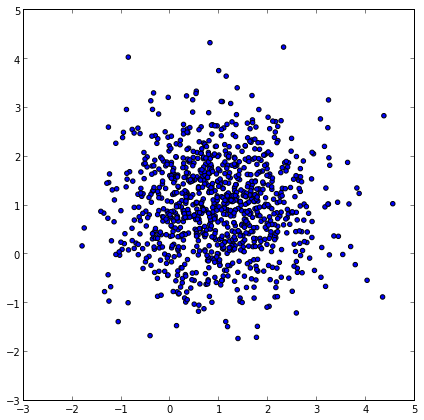# Opposite of zip function in Python

Is there a way to do the opposite of what the zip() function does? It turns out, there is - the zip() function with list unpacking...

### Normal zip() usage

In :
x = [1,2,3,4,5]
y = [10,20,30,40,50]
points = zip(x,y)
points

Out:
[(1, 10), (2, 20), (3, 30), (4, 40), (5, 50)]


### Reverse of normal zip() with list unpacking

By using list unpacking (by using the asterisk before the list), zip can effectively act in reverse...

In :
zip(*points)

Out:
[(1, 2, 3, 4, 5), (10, 20, 30, 40, 50)]


### Example of usefulness

In :
import random
rand = lambda: random.gauss(mu=1, sigma=1)
points = [(rand(), rand()) for i in xrange(1000)]

# Make the graph square
fig = plt.figure()
fig.set_size_inches(7,7)

# The scatter function takes an x-list and a y-list, NOT a list of points
(x, y) = zip(*points)

scatter(x,y)
show()